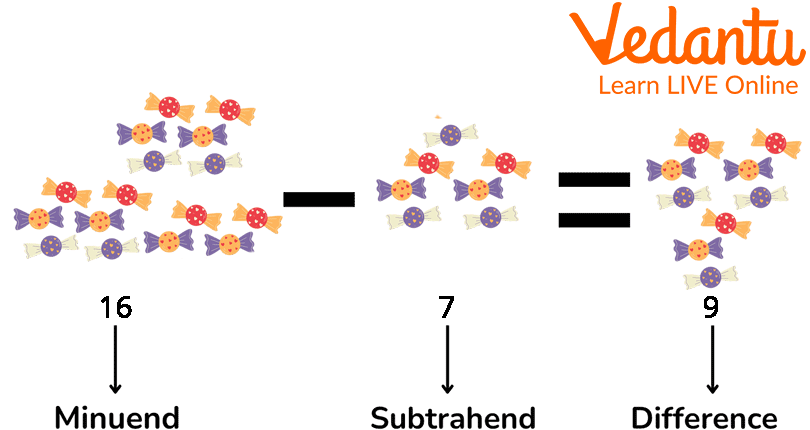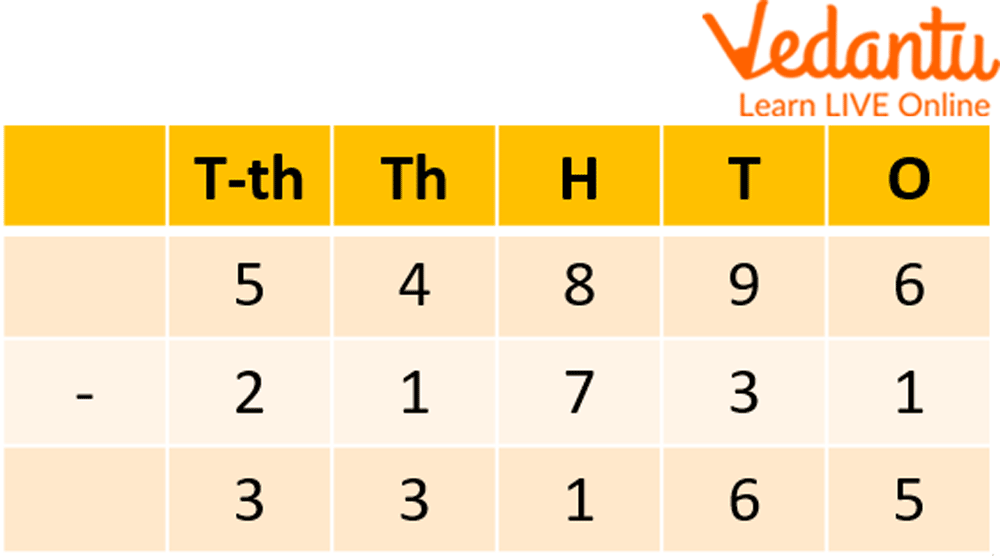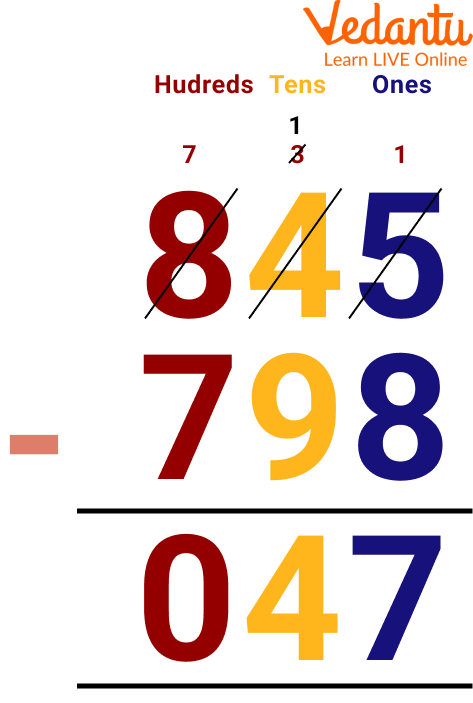Courses
Courses for Kids
Free study material
Free LIVE classes
More

# Rules of Subtraction## Introduction to Subtraction

Subtraction is the process of taking away a number from another. It is a primary arithmetic operation denoted by a subtraction symbol (-) and is the method of calculating the difference between two numbers. The value of a number becomes less when you subtract any number from it. Here we will mainly look at their concepts, examples, and worksheets related to subtraction so that we can understand this article better.

## What is Subtraction?

Subtraction is an operation used to find the difference between numbers. When you have a group of objects and take away a few objects, the group becomes smaller. For example, you bought 9 cupcakes for your birthday party, and your friends ate 7 cupcakes. Now you are left with 2 cupcakes. This can be written as a subtraction expression: 9 - 7 = 2 and is read as "nine minus seven equals two". When we subtract 7 from 9 (9 - 7), we get 2. Here, we performed the subtraction operation on two numbers, 9 and 7, to get the difference of 2.

## Subtraction Formula

When we subtract two numbers, we use some terms which are used in the subtraction expression:

Minuend: The number from which the other number is subtracted.

Subtrahend: The number which is to be subtracted from the minuend.

Difference: The final result after subtracting the subtrahend from the minuend.

The subtraction formula is written as Minuend - Subtrahend = DifferenceSubtraction of Numbers

## How To Solve Subtraction Problems?

While solving subtraction problems, one-digit numbers can be subtracted in a simple way. Still, for larger numbers, we split the numbers into columns using their respective place values, like Ones, Tens, Hundreds, Thousands, and so on. While solving such problems, we may encounter some cases with and some without. Subtraction with borrowing is also known as subtraction with regrouping. When the minuend is smaller than the subtrahend, we use the regrouping method. While regrouping, we borrow 1 number from the preceding column to make the minuend bigger than the subtrahend. Let us understand this with the help of a few examples.

Example: Subtraction of 21731 from 54896

Ans: Given us, Subtraction of 21731 from 54896

Then, After that we will subtract it by placing it in a column, As shown below;Subtraction of Two 5-digit Numbers

## Subtraction Sign Rules

Here, we are going to see adding and subtracting negative numbers rule.

Following are the rules of subtraction of positive and negative numbers:

1. The subtraction of two positive numbers can be either positive or negative.

Example: 5 - 2 = 3, 6 - 8 = -2

1. The subtraction of two negative numbers can be either positive or negative.

Example: (-5) - (-2) = -3, (-5) - (-7) = 2

## Solved Examples

Q 1. Subtraction of 29627 from 76322

Ans: Given us, Subtraction of 29627 from 76322

Then, After that we will subtract it by placing it in a column, As shown below;Subtraction of 29627 and 76322

Q 2. Subtraction of 798 from 845

Ans: Given, Subtraction of 798 from 845

Then, After that we will subtract it by placing it in a column, As shown below;Subtraction of 798 From 845

## Subtraction Practice Problems

Here is a worksheet related to subtraction, which can be improved by practising:

Q 1. 236 - 126

Ans: 110

Q 2. 465 - 237

Ans: 228

Q 3. 126 - 200

Ans: -74

Q 4. 500 - 126

Ans: 374

Q 5. 246 - 186

Ans: 60

## Summary

Subtraction is one of the most important math skills a child needs to learn. It is the foundation for more complicated mathematical concepts such as multiplication and division. Subtraction helps children develop their number sense, which is the understanding that numbers have meaning beyond just a symbol on a page and are necessary for all types of mathematics. Subtraction can help children understand place value, an important concept in all areas of mathematics. It is an important mathematical concept that can solve many problems. In this article, we learned about adding and subtracting negative numbers rules and some problems regarding them.

Last updated date: 26th Sep 2023
Total views: 82.8k
Views today: 2.82k

## FAQs on Rules of Subtraction

1. Where do we use subtraction?

Subtraction is used in our day-to-day life. For example, if we want to know how much money we spent on the items we bought, how much money is left with us, or, if we want to calculate the time left to finish a task, we use subtraction.

2. What are the other words for Subtraction?

The words "Minus", "Less", "Difference", "Decrease", "Take Away", and "Deduct" mean that one number needs to be subtracted from another number.

3. What are some basic important points on subtraction?

Following are some of the important points:

• Any subtraction problem can be transformed into an addition problem and vice-versa.

• Subtracting 0 from any number gives the number itself as the difference.

• When 1 is subtracted from any number, the difference equals the predecessor of the number.